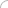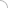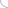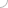# 8086 interview questions and answers

##### 20.Advantages of modular proramming techniques - 8086

Q1. How does the 8086 differ from the 8085 microprocessor?
Q2. Point out the differences between the 8086 and 8088 microprocessor?
Q2. How many bit microprocessor is the 8086 microprocessor?
Q3. Explain the internal architecture of the 8086 microprocessor?
Q4. What is the purpose of the BIU in the 8086?
Q5. Explain the instruction stream queue mechanism in the 8086?
Q6. What does the EU do in the 8086?
Q7. Explain the different modes of operations of the 8086?
Q8. How many I/O ports are present in the 8086?
Q9. What is the significance of the CLK pin in the 8086?
Q10. What is the significance of the NMI pin in the 8086?
Q11. What is the maximum supported clock speed of the 8086?
Q12. What is the purpose of pipe lining in reference to 8086?
Q13. What is the maximum memory addressable size by the 8086?
Q14. State the type of addressing modes supported by the 8086?
Q15. What are the different types of memory addressing modes?
Q16. Explain briefly the string addressing mode with the help of an example?
Q17. State the number and type of registers in the 8086?
Q18. Explain the purpose of the status register?
Q19. What is the purpose of segment register?
Q20. What are the index registers in 8086?
Q21. Define the number of flags present in the 8086 and name each one of them.
Q22. What does the parity flag do?
Q23. What is single stepping and how can it be achieved on the 8086?
Q24. How can interrupts be classified in the 8086?
Q25. Define the process of an interrupt operation in the 8086?
Q26. Define edge or level sensitive interrupts?
Q27. Which interrupt in the 8086 has the highest priority?
Q28. The interrupt response time is determined by?
Q29. What is “daisy-chaining” in reference to the 8086?
Q30. What is the bus arbitration problem in the 8086 and how can it be resolved?
Q31. What type of interrupts are used for critical events and why?
Q32. How can the signals of the 8086 be categorised?
Q33. What is the BHE signal? Explain its significance?
Q34. Describe the AAA instruction with the help of an example?
Q35. Describe the JAE instruction with the help of an example?
Q36. What is the stack segment used for?
Q37. After a pop instruction where does the stack pointer points?
Q38. Define the purpose of SIM and RIM in reference to the 8086?
Q39. Define the functions of the DX register.
Q40. Differentiate between DIV and IDIV instructions with the help of examples.
Q41. What does TASM stand for and what is its use?
Q42. Define what the SOP does with the help of an example.
Q43. Explain with an example how parameters can be passed using registers.
Q44. Define macros with the help of an example.
Q45. State the differences between a procedure and a macro.
Q46. State the differences between absolute and linear select decosing.
Q47. What are the sources of an interrupt?
Q48. Define crossbar switching with reference to 8086?
Q49. With the help of an example explain how physical address is calculated?
Q50. Define PSP and its structure?

 Discussion Board answers thankof 8086 alkuzaay 11-12-2014 12:34 PMInterview questions